### Addition on the Number Line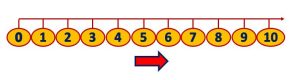Adding a Number on Number Line means we move forward by that number on the Number Line to the Right.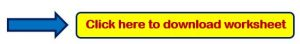## Questions Based on Number Line Addition

Example 1.  Add the Numbers using a Number line?

## 2 + 3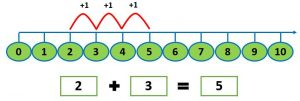Explanation:

Adding means we move forward by 3 numbers to the right on the Number Line, by starting from 2

After moving 3 steps on the number line we would reach 5

### Therefore,  2 + 3 = 5

Example 2.  Add the Numbers using a Number line?

## 31 + 5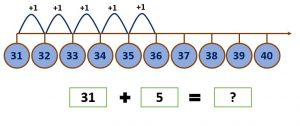Explanation:

Adding 5 means we move forward by 5 numbers to the right on the Number Line, by starting from 31

After moving 5 steps on the number line we would reach 36

### Therefore,  31 + 5 = 36

Example 3.  Add the Numbers using a Number line?

## 4 + 7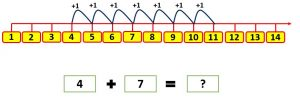Explanation:

Adding 7 means we move forward by 7 numbers to the right on the Number Line, by starting from 4

After moving 7 steps on the number line we would reach 11